# Optical Instruments Questions and Answers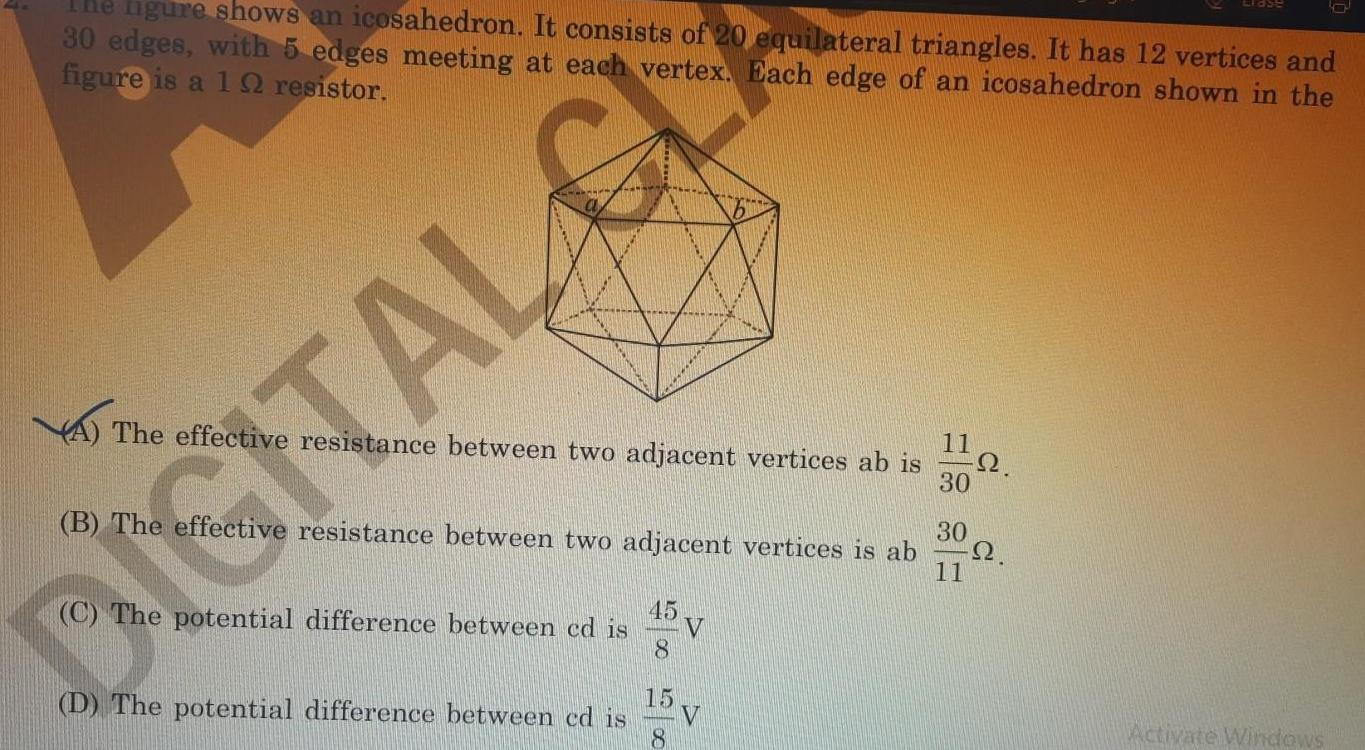Physics
Optical Instruments
ligure shows an icosahedron It consists of 20 equilateral triangles It has 12 vertices and 30 edges with 5 edges meeting at each vertex Each edge of an icosahedron shown in the figure is a 1 2 resistor The effective resistance between two adjacent vertices ab is B The effective resistance between two adjacent vertices is ab DIGITAL G 45 C The potential difference between cd is 8 15 D The potential difference between cd is 8 V V 11 30 30 11 2 2 Activate Windows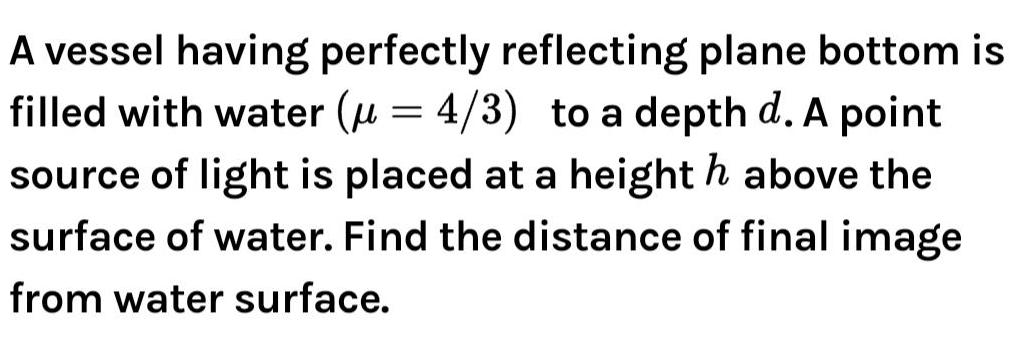Physics
Optical Instruments
A vessel having perfectly reflecting plane bottom is filled with water 4 3 to a depth d A point source of light is placed at a height h above the surface of water Find the distance of final image from water surface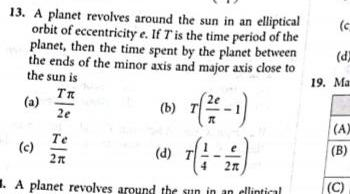Physics
Optical Instruments
13 A planet revolves around the sun in an elliptical orbit of eccentricity e If T is the time period of the planet then the time spent by the planet between the ends of the minor axis and major axis close to the sun is a c 58 25 TR 2e Te b 7 7 7 1 d 4 3 2n A planet revolves around the sun in an elliptical c d 19 Ma A B C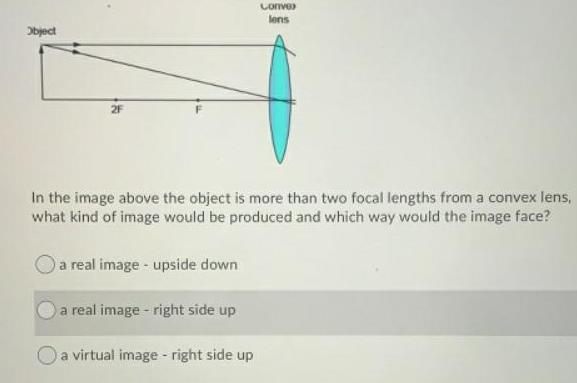Physics
Optical Instruments
Object Conve lens In the image above the object is more than two focal lengths from a convex lens what kind of image would be produced and which way would the image face a real image upside down a real image right side up a virtual image right side up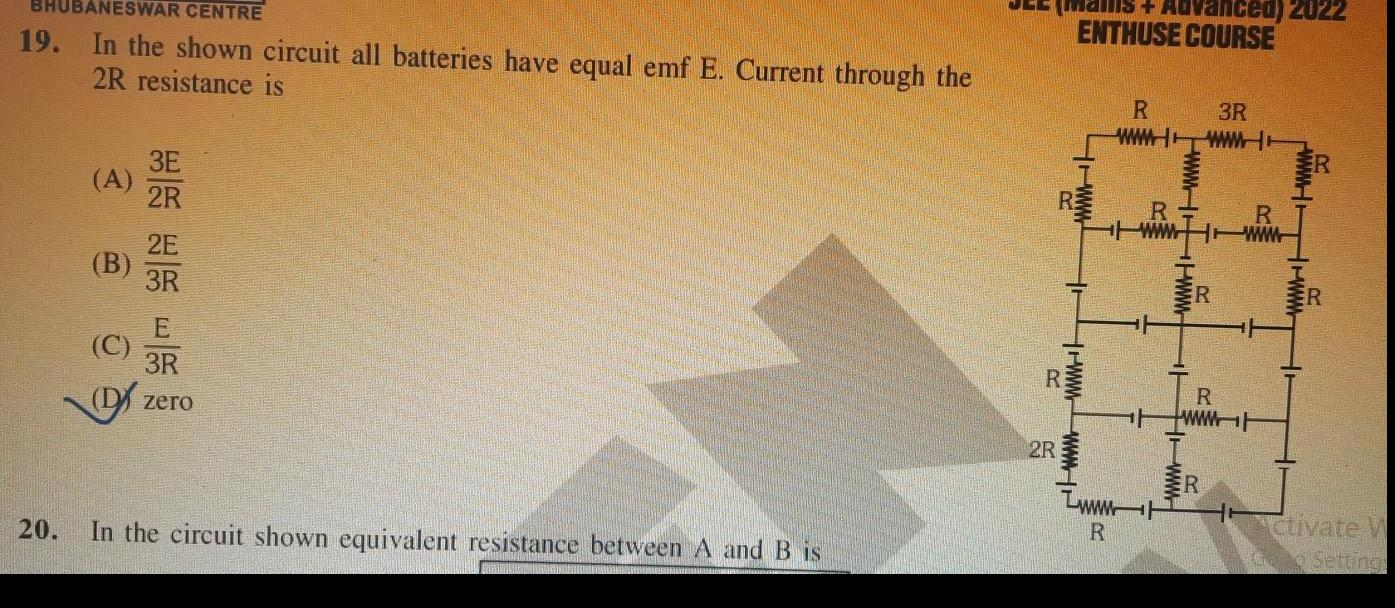Physics
Optical Instruments
BANESWAR CENTRE 19 In the shown circuit all batteries have equal emf E Current through the 2R resistance is B 60 3E 2R 3R zero 20 In the circuit shown equivalent resistance between A and B is JEE Mai Advanced 2022 ENTHUSE COURSE Z 2R wwwwwwww www R R wwwwwwwww R 3R wwwww wwwwwww R R www www Ctivate V o Settings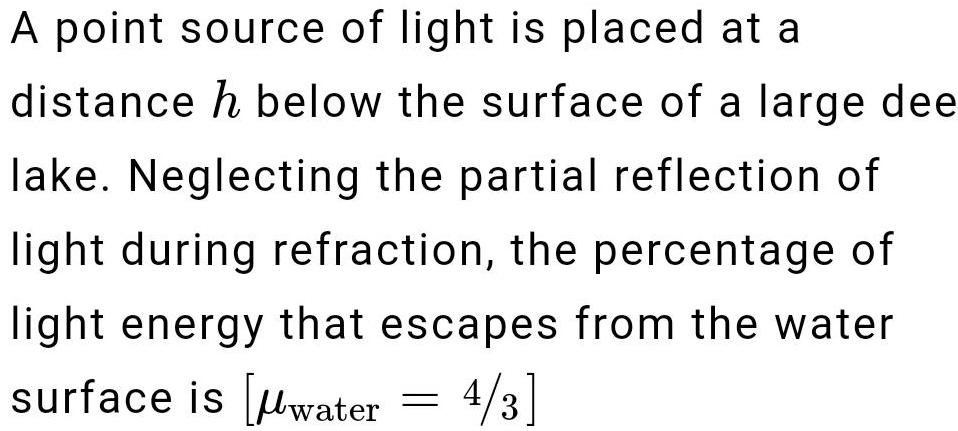Physics
Optical Instruments
A point source of light is placed at a distance h below the surface of a large dee lake Neglecting the partial reflection of light during refraction the percentage of light energy that escapes from the water surface is water 4 3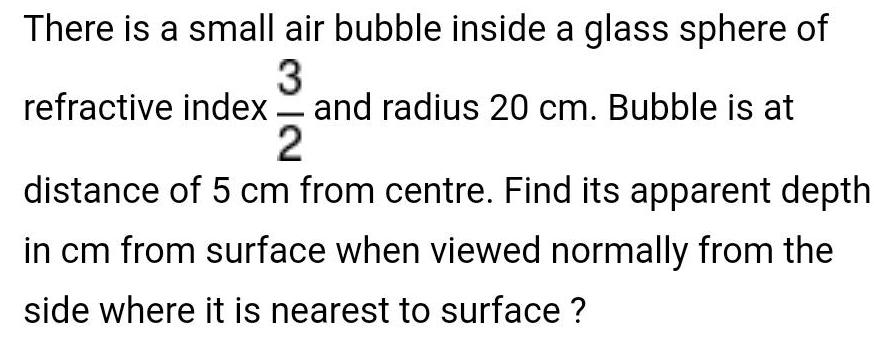Physics
Optical Instruments
There is a small air bubble inside a glass sphere of 3 refractive index and radius 20 cm Bubble is at 2 distance of 5 cm from centre Find its apparent depth in cm from surface when viewed normally from the side where it is nearest to surface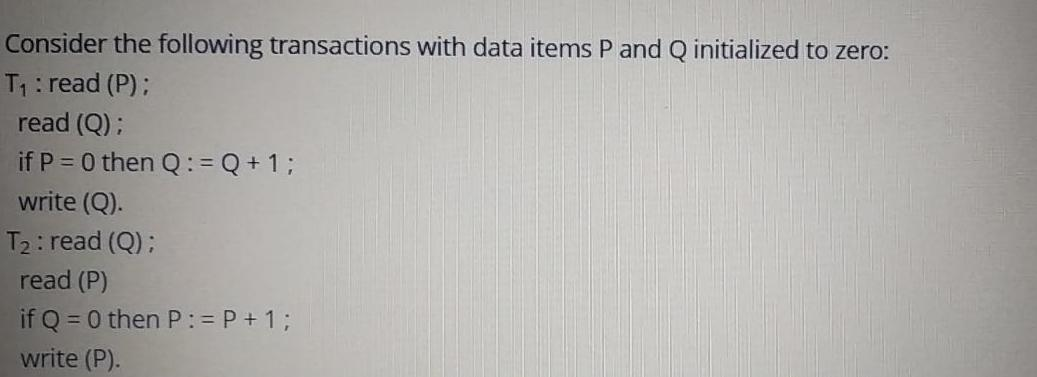Physics
Optical Instruments
Consider the following transactions with data items P and Q initialized to zero T read P read Q if P 0 then Q Q 1 write Q T read Q read P if Q 0 then P P 1 write P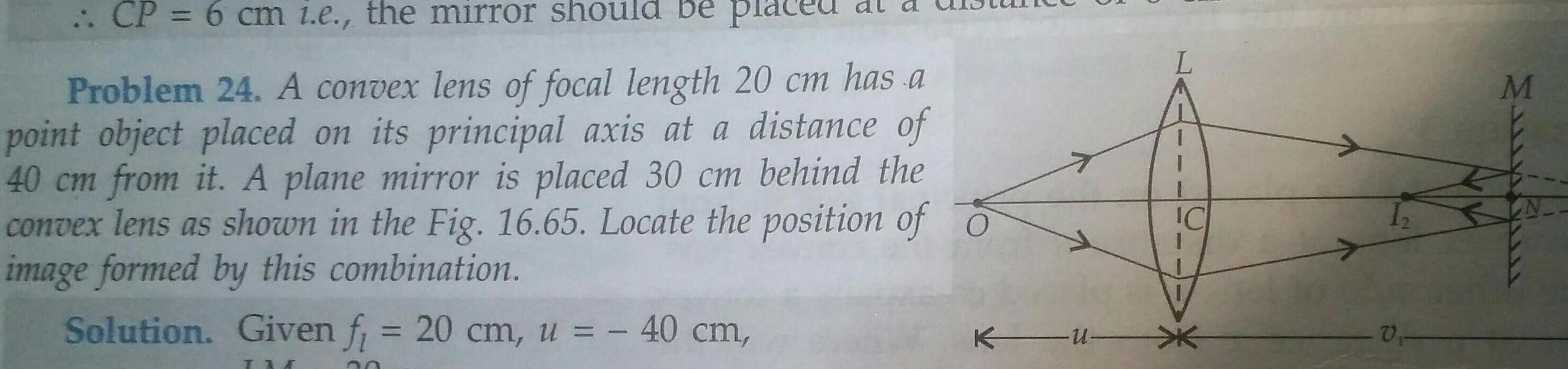Physics
Optical Instruments
CP 6 cm i e the mirror should be pla Problem 24 A convex lens of focal length 20 cm has a point object placed on its principal axis at a distance of 40 cm from it A plane mirror is placed 30 cm behind the convex lens as shown in the Fig 16 65 Locate the position of o image formed by this combination Solution Given f 20 cm u 40 cm IM KU IC 12 V M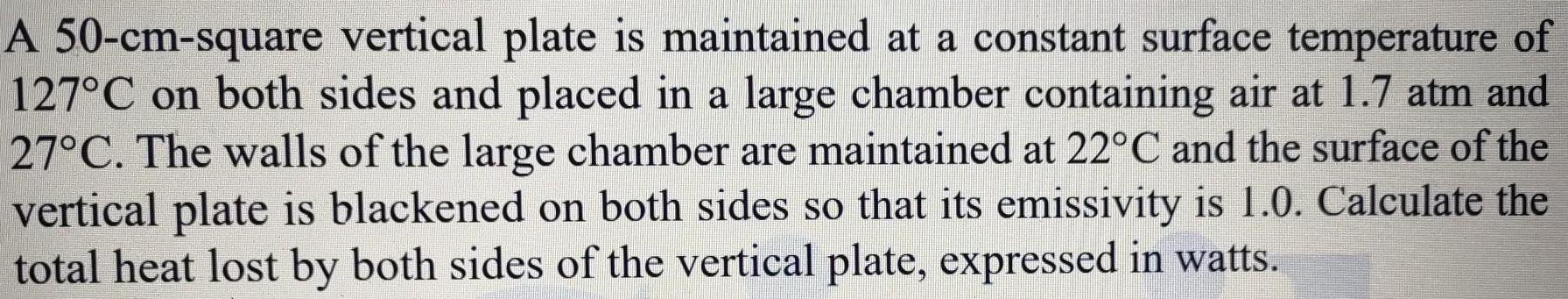Physics
Optical Instruments
A 50 cm square vertical plate is maintained at a constant surface temperature of 127 C on both sides and placed in a large chamber containing air at 1 7 atm and 27 C The walls of the large chamber are maintained at 22 C and the surface of the vertical plate is blackened on both sides so that its emissivity is 1 0 Calculate the total heat lost by both sides of the vertical plate expressed in watts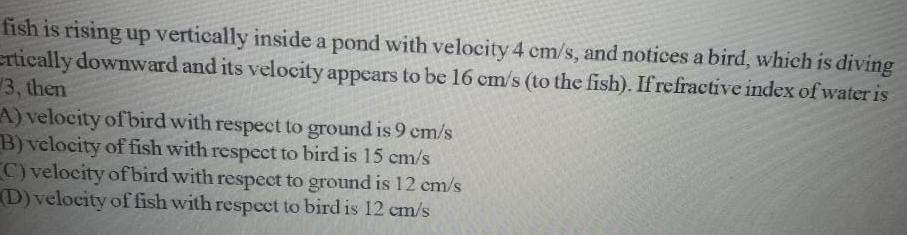Physics
Optical Instruments
fish is rising up vertically inside a pond with velocity 4 cm s and notices a bird which is diving ertically downward and its velocity appears to be 16 cm s to the fish If refractive index of water is 3 then A velocity of bird with respect to ground is 9 cm s B velocity of fish with respect to bird is 15 cm s C velocity of bird with respect to ground is 12 cm s D velocity of fish with respect to bird is 12 cm s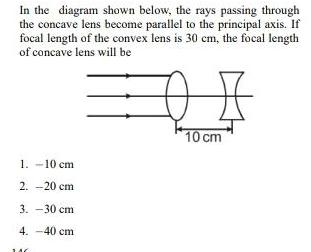Physics
Optical Instruments
In the diagram shown below the rays passing through the concave lens become parallel to the principal axis If focal length of the convex lens is 30 cm the focal length of concave lens will be 1 10 cm 2 20 cm 3 30 cm 4 40 cm B 10 cm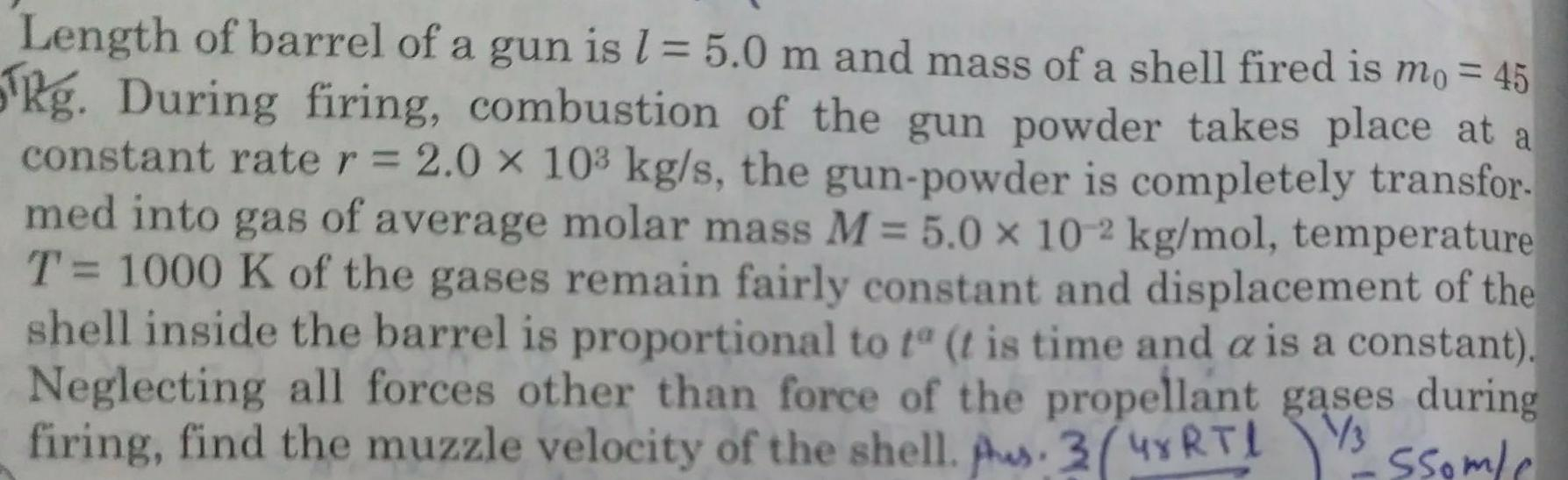Physics
Optical Instruments
Length of barrel of a gun is l 5 0 m and mass of a shell fired is mo 45 Rg During firing combustion of the gun powder takes place at a constant rate r 2 0 x 10 kg s the gun powder is completely transfor med into gas of average molar mass M 5 0 x 10 2 kg mol temperature T 1000 K of the gases remain fairly constant and displacement of the shell inside the barrel is proportional to ta t is time and a is a constant Neglecting all forces other than force of the propellant gases during firing find the muzzle velocity of the shell Aus 3 4 R T l 1 3 issomle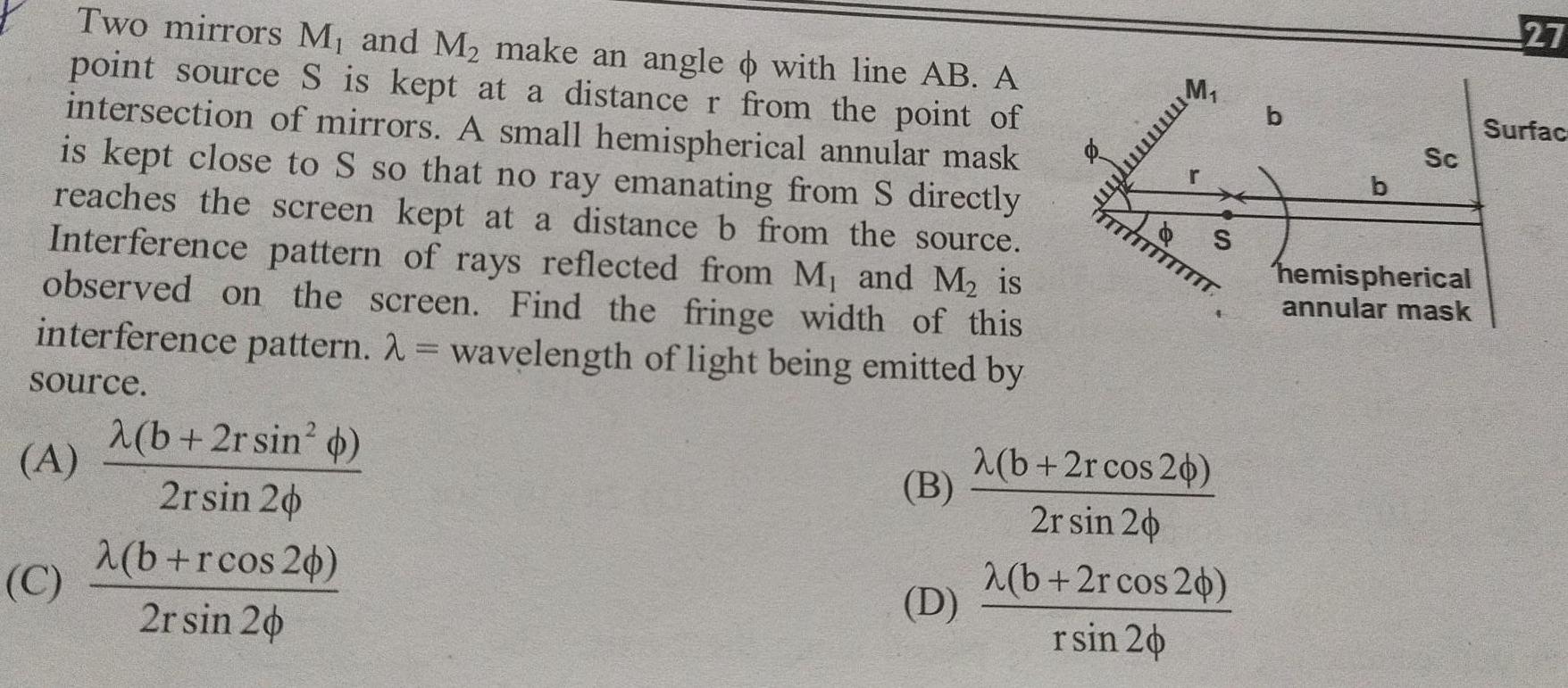Physics
Optical Instruments
Two mirrors M and M make an angle with line AB A point source S is kept at a distance r from the point of intersection of mirrors A small hemispherical annular mask is kept close to S so that no ray emanating from S directly reaches the screen kept at a distance b from the source Interference pattern of rays reflected from M and M is observed on the screen Find the fringe width of this interference pattern wavelength of light being emitted by source A C 2 b 2rsin b 2rsin 20 b rcos 20 2r sin 20 B D M m S b 2r cos 26 2r sin 20 2 b 2rcos 20 rsin 20 b b Sc hemispherical annular mask 27 Surfac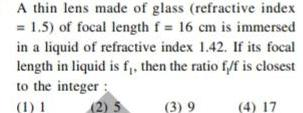Physics
Optical Instruments
A thin lens made of glass refractive index 1 5 of focal length f 16 cm is immersed in a liquid of refractive index 1 42 If its focal length in liquid is f then the ratio f f is closest to the integer 1 1 2 5 3 9 4 17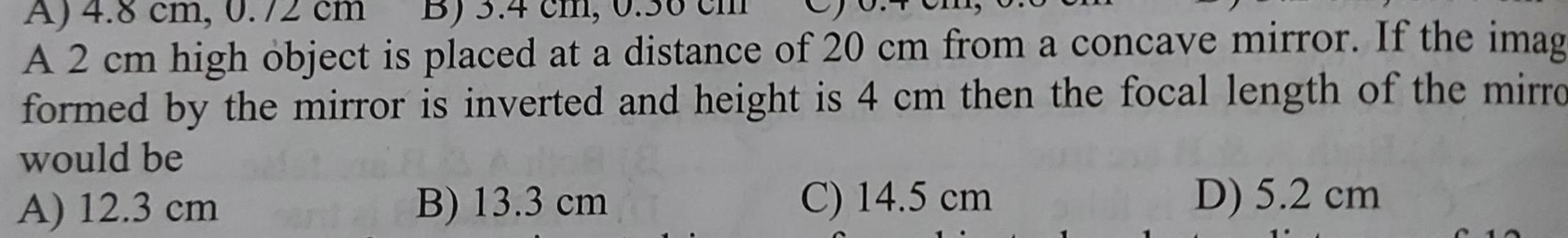Physics
Optical Instruments
A 4 8 cm B 3 4 A 2 cm high object is placed at a distance of 20 cm from a concave mirror If the imag formed by the mirror is inverted and height is 4 cm then the focal length of the mirro would be A 12 3 cm B 13 3 cm C 14 5 cm D 5 2 cm C 1